# Determinant of a Matrix## Determinant of a Matrix

Determinants originally appeared in the study of the linear equations. We shall, however, associate the notion of the determinant with matrices. For this purpose, we have to consider the square matrices of order 1, 2, 3 etc.

********************

10 Math Problems officially announces the release of Quick Math Solver, an Android App on the Google Play Store for students around the world.

********************

If A is a square matrix, its determinant is denoted by |A| or det.(A). And, the value of determinant is calculated differently for different ordered square matrices. Here is mentioned about the calculation of determinants of square matrices of order 1, 2 and 3.

### Determinant of a 1×1 Matrix

Let A = (a11) be a square matrix of order 1×1. Then, its determinant |A| is given simply by the value of its single element a11.

i.e. |A| = |a11| = a11

For example: Let, A = (2), then

Determinant of A = |A| = |2| = 2

### Determinant of a 2×2 Matrix### Determinant of a 3×3 MatrixHere is an easy pattern to find the minors of any element of a 3×3 matrix:Note: Determinant of 3×3 matrix A can also be calculated by taking the products of the elements of any row (or column) and the corresponding cofactors. i.e.

|A| = a11A11 + a12A12 + a13A13

Or,

|A| = a21A21 + a22A22 + a23A23

Or,

|A| = a31A31 + a32A32 + a33A33

Or,

|A| = a11A11 + a21A21 + a31A31

Or,

|A| = a12A12 + a22A22 + a32A32

Or,

|A| = a13A13 + a23A23 + a33A33

All will give the same value for the determinant of A.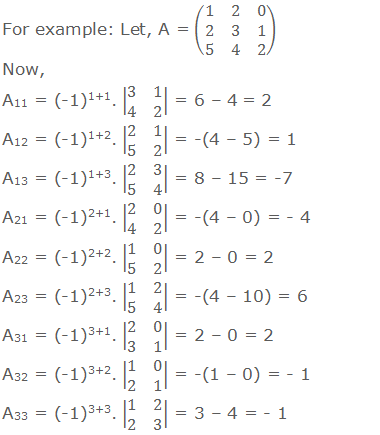Now,

|A| = a11A11 + a12A12 + a13A13 = 1×2 + 2×1 + 0×-7 = 4

|A| = a21A21 + a22A22 + a23A23 = 2×-4 + 3×2 + 1×6 = 4

|A| = a31A31 + a32A32 + a33A33 = 5×2 + 4×-1 + 2×-1 = 4

|A| = a11A11 + a21A21 + a31A31 = 1×2 + 2×-4 + 5×2 = 4

|A| = a12A12 + a22A22 + a32A32 = 2×1 + 3×2 + 4×-1 = 4

|A| = a13A13 + a23A23 + a33A33 = 0×-7 + 1×6 + 2×-1 = 4

Here, we got |A| = 4 in every calculations, so we can use any one of the above methods. Generally, we use the first method to calculate the determinant of a 3×3 matrix A. i.e.

|A| = a11A11 + a12A12 + a13A13

### Mechanical Rule (Rule of Sarrus)

A mechanical rule for finding the value of a third order determinant is as indicated below:-From the algebraic sum of the products of the elements of the diagonals pointing downwards, subtract the algebraic sum of the products of the elements of the diagonals pointing upwards.

This rule of expansion of a third-order determinant is known as The Rule of Sarrus.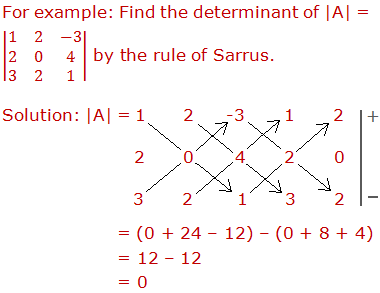Note: This rule does not work for the determinants of the order greater than 3.

### Properties of 3×3 Determinant

We have seen how to evaluate a 3×3 determinant. There are some properties of 3×3 determinant. Look at them and their verification.

Prop. 1: The value of the determinant is unaltered by interchanging its rows and columns.

Verification: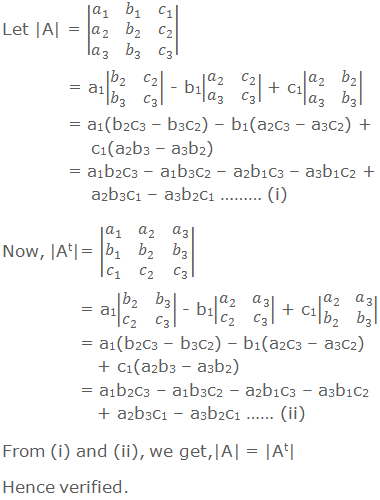Prop. 2: Interchanging any two adjacent rows (or columns) changes the sign of the determinant.

Verification: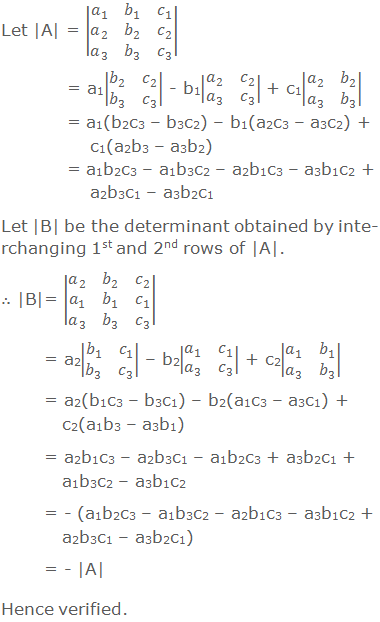Prop. 3: If any two rows (or columns) of a determinant are identical, then the value of the determinant is zero.

Verification:Prop. 4: If all the elements of any row (or column) are multiplied by a constant k, then the value of the determinant is multiplied by k.

Verification: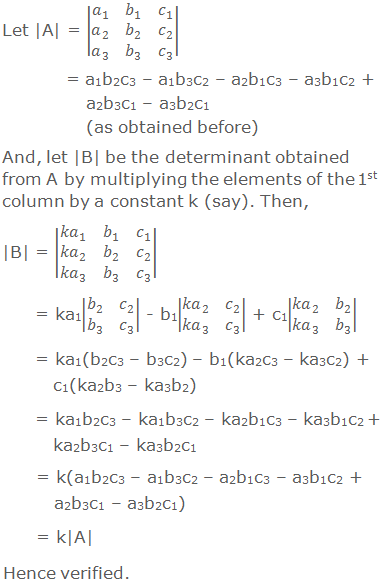Prop. 5: If each element of any row (or column) of a determinant is expressed as the sum of two terms, then the determinant can be expressed as the sum of determinants.

Verification:Prop. 6: If to the elements of any row (or column) a multiple of any other row (or column) is added, the value of the determinant remains unaltered.

Verification: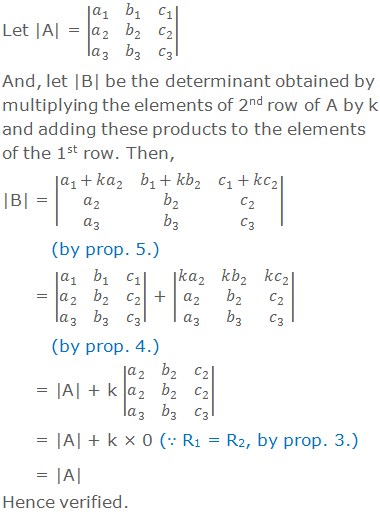### Worked Out Examples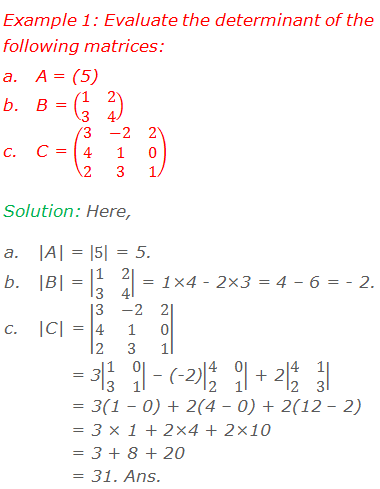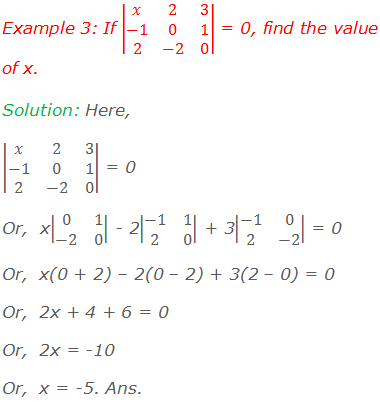#### Do you have any questions regarding the determinant of a matrix?

You can ask your questions or problems here, in the comment section below.